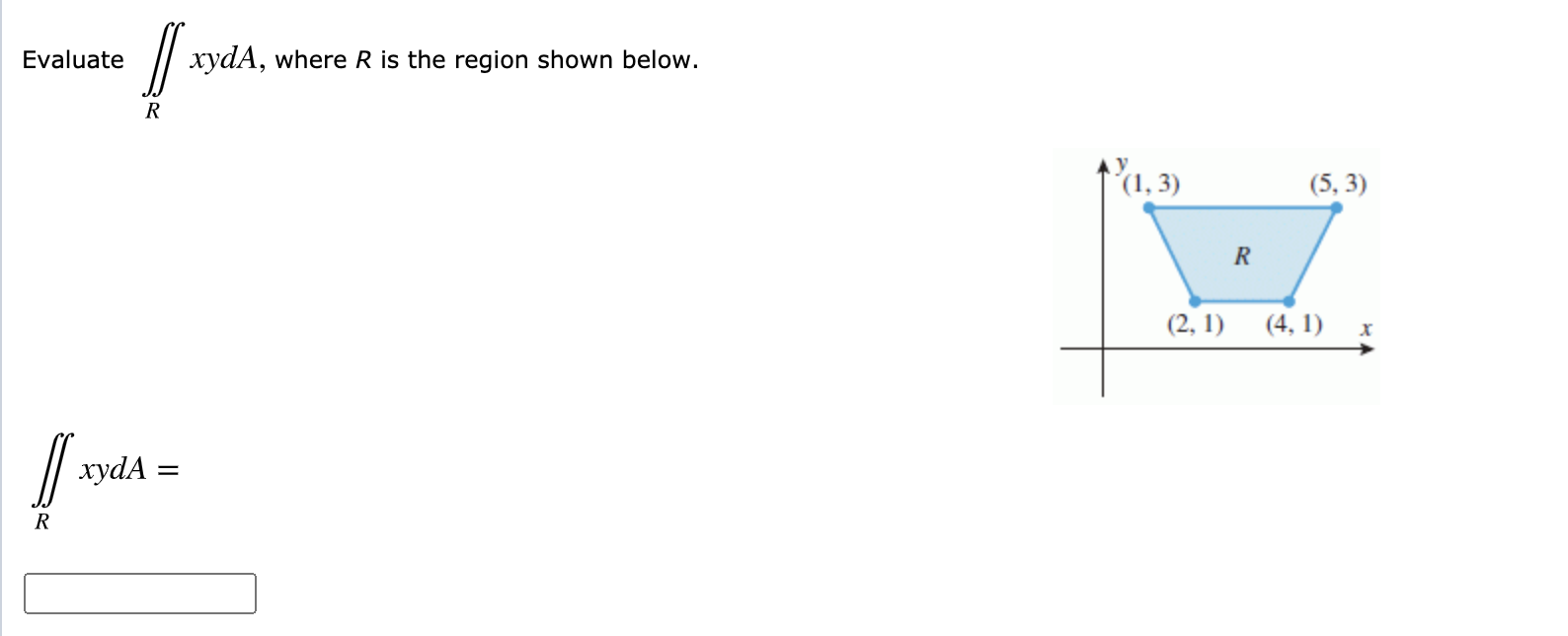# Question Solved1 AnswerEvaluate ∬RxydA, where R is the region shown below. Evaluate xydA, where R is the region shown below. R (1,3) (5,3) R (2, 1) (4,1) x xydAEvaluate ∬RxydA, where R is the region shown below.

Transcribed Image Text: Evaluate xydA, where R is the region shown below. R (1,3) (5,3) R (2, 1) (4,1) x xydA
More
Transcribed Image Text: Evaluate xydA, where R is the region shown below. R (1,3) (5,3) R (2, 1) (4,1) x xydA